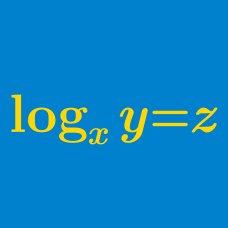Algebra

# Logarithmic Functions: Level 2 Challenges

Evaluate the following:

$\Large 9^{\log_{3}(4)}$

Do not use a calculator -- not even a slide rule!

$\large \log_2 3 \times \log_3 4 \times \log_4 5 \times \cdots\times \log_{127} 128 = \, ?$

True or false:

$\quad \quad$ $\log_{x}1=0$ for every $x>0$.

$\large \sum_{\text{cyclic}(a,b,c)} \left( \dfrac1{1 + \log_a bc} \right) = \, ?$

Which of the options is correct about the number $\log _{ 4 }{ 18 }$?

×